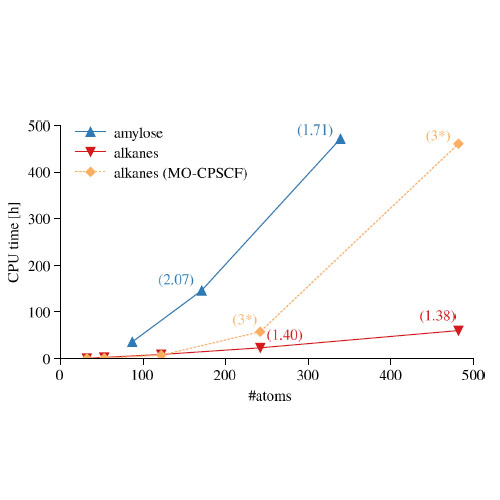## A reduced-scaling density matrix-based method for the computation of the vibrational Hessian matrix at the self-consistent field level

J. Chem. Phys., 142, 094101, http://dx.doi.org/10.1063/1.4908131
J. Chem. Phys., online article

An analytical method to calculate the molecular vibrational Hessian matrix at the self-consistent field level is presented. By analysis of the multipole expansions of the relevant derivatives of Coulomb-type two-electron integral contractions, we show that the effect of the perturbation on the electronic structure due to the displacement of nuclei decays at least as r −2 instead of r −1. The perturbation is asymptotically local, and the computation of the Hessian matrix can, in principle, be performed with O(N) complexity. Our implementation exhibits linear scaling in all time-determining steps, with some rapid but quadratic-complexity steps remaining. Sample calculations illustrate linear or near-linear scaling in the construction of the complete nuclear Hessian matrix for sparse systems. For more demanding systems, scaling is still considerably sub-quadratic to quadratic, depending on the density of the underlying electronic structure.